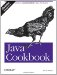# Recipe 5.5 Ensuring the Accuracy of Floating-Point Numbers

#### Problem

You want to know if a floating-point computation generated a sensible result.

#### Solution

Compare with the INFINITY constants, and use isNaN( ) to check for "not a number."

Fixed-point operations that can do things like divide by zero result in Java notifying you abruptly by throwing an exception. This is because integer division by zero is considered a logic error.

Floating-point operations, however, do not throw an exception because they are defined over an (almost) infinite range of values. Instead, they signal errors by producing the constant POSITIVE_INFINITY if you divide a positive floating-point number by zero, the constant NEGATIVE_INFINITY if you divide a negative floating-point value by zero, and NaN (Not a Number), if you otherwise generate an invalid result. Values for these three public constants are defined in both the Float and the Double wrapper classes. The value NaN has the unusual property that it is not equal to itself, that is, NaN != NaN. Thus, it would hardly make sense to compare a (possibly suspect) number against NaN, because the expression:

`x == NaN`

can never be true. Instead, the methods Float.isNaN(float) and Double.isNaN(double) must be used:

`// InfNaN.java  public static void main(String argv[]) {      double d = 123;      double e = 0;      if (d/e == Double.POSITIVE_INFINITY)          System.out.println("Check for POSITIVE_INFINITY works");      double s = Math.sqrt(-1);      if (s == Double.NaN)          System.out.println("Comparison with NaN incorrectly returns true");      if (Double.isNaN(s))          System.out.println("Double.isNaN( ) correctly returns true");  }`

Note that this, by itself, is not sufficient to ensure that floating-point calculations have been done with adequate accuracy. For example, the following program demonstrates a contrived calculation Heron's formula for the area of a triangle both in float and in double. The double values are correct, but the floating-point value comes out as zero due to rounding errors. This happens because, in Java, operations involving only float values are performed as 32-bit calculations. Related languages such as C automatically promote these to double during the computation, which can eliminate some loss of accuracy.

`/** Compute the area of a triangle using Heron's Formula.  * Code and values from Prof W. Kahan and Joseph D. Darcy.  * See http://www.cs.berkeley.edu/~wkahan/JAVAhurt.pdf.  * Derived from listing in Rick Grehan's Java Pro article (October 1999).  * Simplified and reformatted by Ian Darwin.  */ public class Heron {     public static void main(String[] args) {         // Sides for triangle in float         float af, bf, cf;         float sf, areaf;         // Ditto in double         double ad, bd, cd;         double sd, aread;         // Area of triangle in float         af = 12345679.0f;         bf = 12345678.0f;         cf = 1.01233995f;         sf = (af+bf+cf)/2.0f;         areaf = (float)Math.sqrt(sf * (sf - af) * (sf - bf) * (sf - cf));         System.out.println("Single precision: " + areaf);         // Area of triangle in double         ad = 12345679.0;         bd = 12345678.0;         cd = 1.01233995;         sd = (ad+bd+cd)/2.0d;         aread =        Math.sqrt(sd * (sd - ad) * (sd - bd) * (sd - cd));         System.out.println("Double precision: " + aread);     } }`

Let's run it. To ensure that the rounding is not an implementation artifact, I'll try it both with Sun's JDK and with Kaffe:

`\$ java Heron Single precision: 0.0 Double precision: 972730.0557076167 \$ kaffe Heron Single precision: 0.0 Double precision: 972730.05570761673`

If in doubt, use double!

To ensure consistency of very large magnitude double computations on different Java implementations, Java provides the keyword strictfp , which can apply to classes, interfaces, or methods within a class. If a computation is Strict-FP, then it must always, for example, return the value INFINITY if a calculation would overflow the value of Double.MAX_VALUE (or underflow the value Double.MIN_VALUE). Non-Strict-FP calculations the default are allowed to perform calculations on a greater range and can return a valid final result that is in range even if the interim product was out of range. This is pretty esoteric and affects only computations that approach the bounds of what fits into a double.

 Note that an expression consisting entirely of compile-time constants, like Math.PI * 2.1e17, is also considered to be Strict-FP.Java Cookbook, Second Edition
ISBN: 0596007019
EAN: 2147483647
Year: 2003
Pages: 409
Authors: Ian F Darwin

Similar book on Amazon